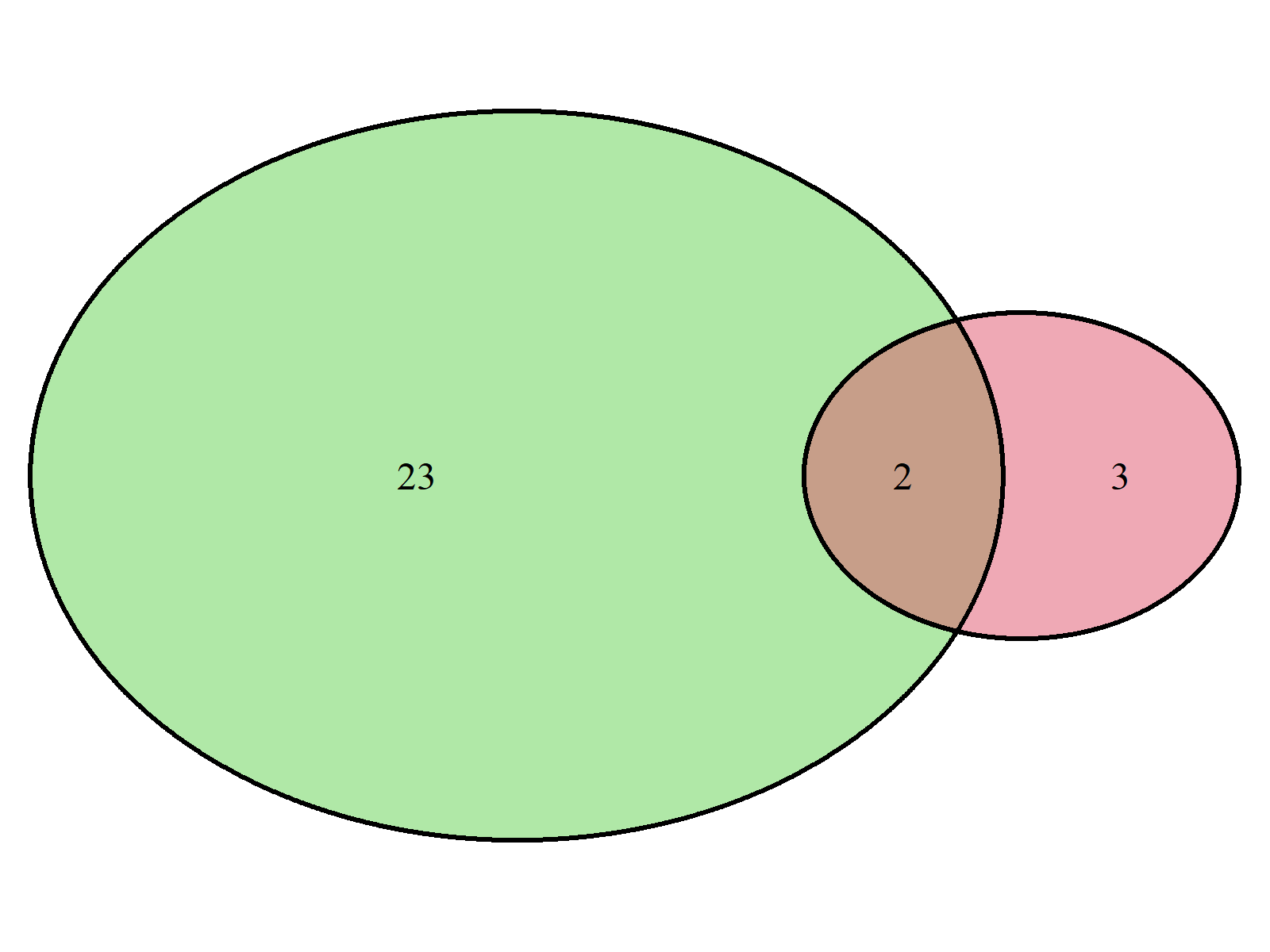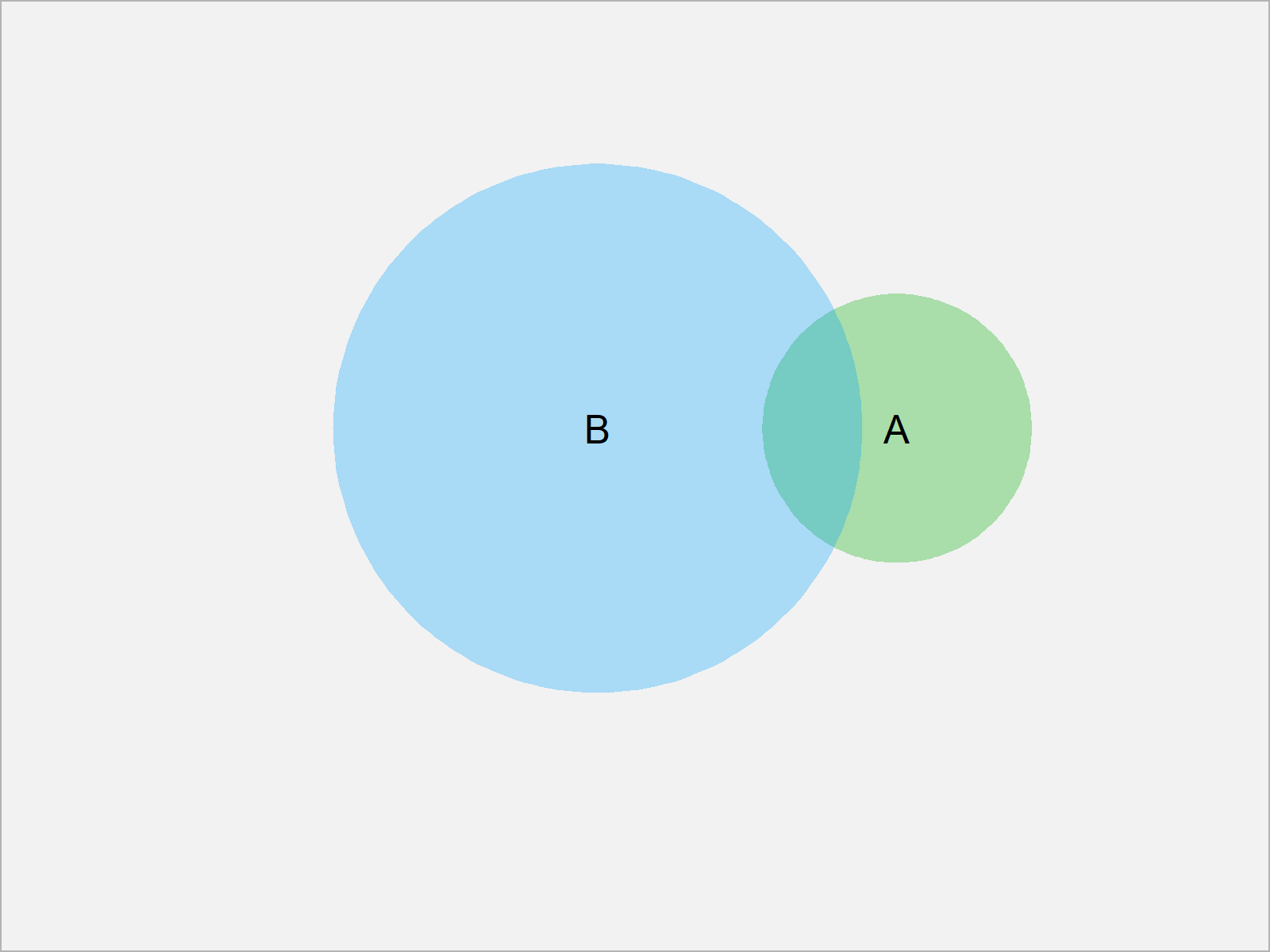# Venn Diagram with Opacity in R (2 Examples)

In this R tutorial you’ll learn how to create a venn diagram with transparent colors.

It’s time to dive into the exemplifying R syntax.

## Example 1: Venn Diagram with Transparent Color Shading Using VennDiagram Package

Example 1 explains how to create a transparent venn diagram using the VennDiagram package.

We first need to install and load the VennDiagram package, if we want to use the functions that are contained in the add-on package:

```install.packages("VennDiagram")  # Install VennDiagram package

Next, we can draw a colored venn diagram with opacity as shown below:

```grid.newpage()                   # Create new plotting page
draw.pairwise.venn(area1 = 5,    # Draw pairwise venn diagram
area2 = 25,
cross.area = 2,
fill = 2:3)```The venn diagram we have created with the previous R code is shown in Figure 1. As you can see, both circles are colored and the overlapping area is transparent.

## Example 2: Venn Diagram with Transparent Color Shading Using venneuler Package

This example explains how to use the venneuler package to create a venn diagram with lowered alpha value.

First, we need to install and load the venneuler package:

```install.packages("venneuler")    # Install & load venneuler
library("venneuler")```

Now, we can apply the venneuler function in combination with the plot function as shown below:

```plot(venneuler(c(A = 5,          # Draw pairwise venn diagram
B = 25,
"A&B" = 2)))```As shown in Figure 1, the previous R syntax has plotted a venn diagram with transparent colors.

## Video, Further Resources & Summary

I have recently published a video on my YouTube channel, which explains the R syntax of this tutorial. You can find the video below.

Please accept YouTube cookies to play this video. By accepting you will be accessing content from YouTube, a service provided by an external third party.If you accept this notice, your choice will be saved and the page will refresh.

Furthermore, you may want to read the related articles of this website. Some tutorials about graphics in R can be found below.

In summary: In this article, I have explained how to draw transparent venn diagrams in the R programming language. If you have further questions, let me know in the comments.

Subscribe to the Statistics Globe Newsletter